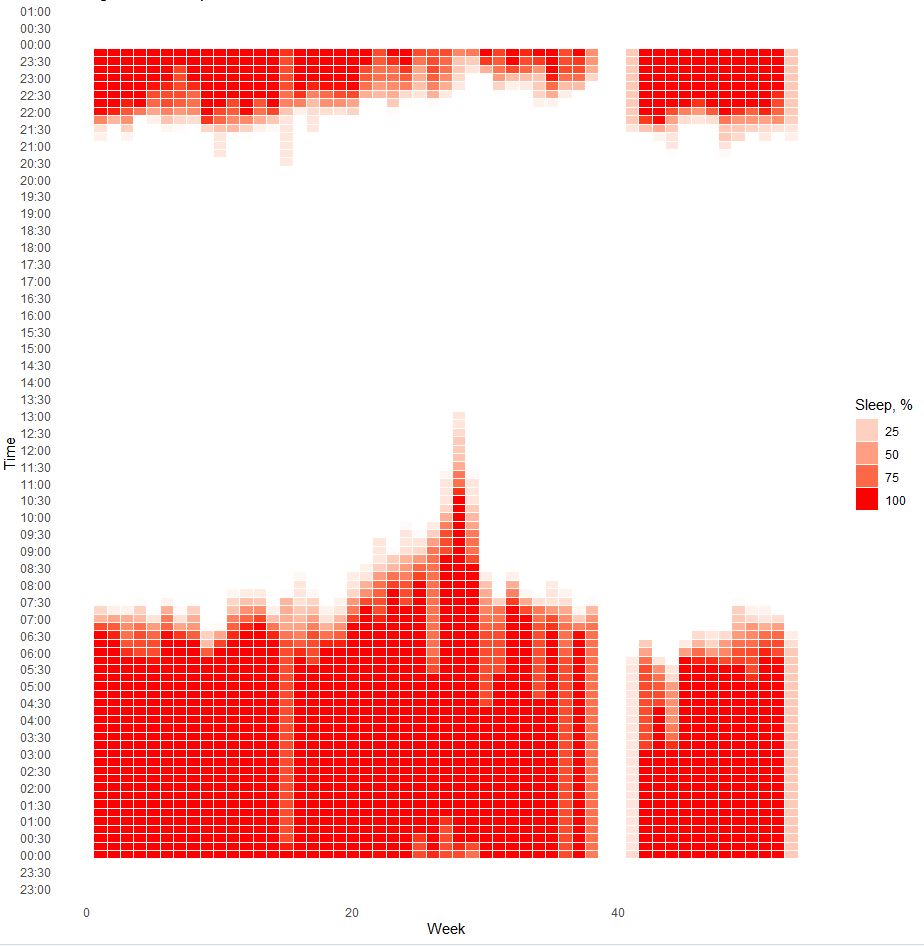Quantified Self Experiments / Data Visualization - Sleep schedule

Strict sleep schedule seems to be important to have lower sleep onset latency, better sleep quality and keeping our circadian clock in sync..There is good explanations of scientific background in a Why we sleep book by Matthew Walker.

Since i have detailed sleep tracking data, i can check what is my usual sleep schedule.

Heatmap of sleep status versus time fo day:X - week number, Y - time of day, Color - % percentage of sleep per time period.

As we can see my usual bedtime start is between 22:00 and 22:45 and morning rise time is between 6:30 and 7:00. These timings confirms my constant sleep schedule. Acute increase in rising time at weeks 28-32 were due to COVID-19.

Data is available here.

`library(lubridate)library(dplyr)library(plyr)library(ggplot2)#sleep status for 30 sec periodsdf <- read.csv("https://blog.kto.to/uploads/sleep-status.csv") df <- df[!is.na(df\$`SleepStatus`),];df\$ymd <-ymd_hms(df\$datetime)df\$date <-as.Date(df\$ymd)df\$month <- month(df\$datetime, label = TRUE)df\$year <- year(df\$datetime)df\$day <- day(df\$datetime)df\$week <- week(df\$datetime)df\$wday <- wday(df\$datetime, label = TRUE)df\$hour <- hour(df\$datetime)df\$minute <- 60*60*df\$hour + round(minute(df\$datetime)/15)*15*60df\$time <- format(as.POSIXct('1900-1-1') + df\$minute, '%H:%M:%S')hdf <- ddply(df, c( "time", "week"), summarise, SleepStatus = 100*sum(SleepStatus)/(7*2*15))hdf\$x <- hdf\$week#hdf\$x <- factor(hdf\$month, levels=rev(levels(hdf\$month)))hdf\$y <- as.POSIXct(strptime(hdf\$time, format = "%H:%M:%S"))hdf\$f <- hdf\$SleepStatuslibrary(scales)ggplot(hdf, aes(x, y)) + geom_tile(aes(fill = f), colour = "white", na.rm = TRUE) +  scale_fill_gradient(low = "white", high = "red") +    guides(fill=guide_legend(title="Sleep, %")) +  scale_y_datetime(date_breaks = "30 min", date_labels = "%H:%M") +  theme_bw() + theme_minimal() +   labs(title = "Histogram of Sleep", x = "Week", y = "Time") +  theme(panel.grid.major = element_blank(), panel.grid.minor = element_blank())`

#### Statistical analysis

RStudio version 1.3.959 and R version 4.0.2.